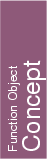## Kernel::ConstructCircle_2

A model for this must provide:

Kernel::Circle_2
 fo.operator() ( Kernel::Point_2 center, Kernel::FT squared_radius, Orientation orientation = COUNTERCLOCKWISE)
introduces a variable of type Kernel::Circle_2. It is initialized to the circle with center center, squared radius squared_radius and orientation orientation.
Precondition: orientationCOLLINEAR, and further, squared_radius0.

Kernel::Circle_2
 fo.operator() ( Kernel::Point_2 p, Kernel::Point_2 q, Kernel::Point_2 r)
introduces a variable of type Kernel::Circle_2. It is initialized to the unique circle which passes through the points p, q and r. The orientation of the circle is the orientation of the point triple p, q, r.
Precondition: p, q, and r are not collinear.

Kernel::Circle_2
 fo.operator() ( Kernel::Point_2 p, Kernel::Point_2 q, Orientation orientation = COUNTERCLOCKWISE)
introduces a variable of type Kernel::Circle_2. It is initialized to the circle with diameter pq and orientation orientation.
Precondition: orientationCOLLINEAR.

Kernel::Circle_2
 fo.operator() ( Kernel::Point_2 center, Orientation orientation = COUNTERCLOCKWISE)
introduces a variable of type Kernel::Circle_2. It is initialized to the circle with center center, squared radius zero and orientation orientation.
Precondition: orientationCOLLINEAR.
Postcondition: .is_degenerate() = true.